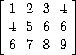# Matrix operations

Suppose we represent vectors `v = (vᵢ)` as sequences of numbers, and matrices `m = (mᵢⱼ)` as sequences of vectors (the rows of the matrix). For example, the matrixis represented as the sequence `((1 2 3 4) (4 5 6 6) (6 7 8 9))` . With this representation, we can use sequence operations to concisely express the basic matrix and vector operations. These operations (which are described in any book on matrix algebra) are the following:

`(dot-product v w)` returns the sum `∑ᵢvᵢwᵢ`

`(matrix-*-vector m v)` returns the vector `t` , where `tᵢ = ∑ⱼmᵢⱼvᵢ`

`(matrix-*-matrix m n)` returns the matrix `p` , where `pᵢⱼ = ∑ₖmᵢₖnₖⱼ`

`(transpose m)` returns the matrix `n` , where `nᵢⱼ = mⱼᵢ`

We can define the dot product as

``````(define (dot-product v w)
(accumulate + 0 (map * v w)))
``````

Fill in the missing expressions in the following procedures for computing the other matrix operations. (The procedure `accumulate-n` is defined in exercise 2.36 .)

``````(define (matrix-*-vector m v)
(map <??> m))
(define (transpose mat)
(accumulate-n <??> <??> mat))
(define (matrix-*-matrix m n)
(let ((cols (transpose n)))
(map <??> m)))
``````